# The formula for a Bezier curve

Suppose we have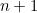control points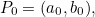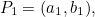...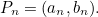We will represent the corresponding Bézier curve by points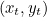whereruns from 0 to 1. In other words, for eachbetween 0 and 1 we get a pointand together these points form the curve. The formulae for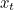and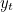are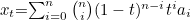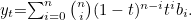where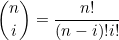is the binomial coefficient.

Back to main article

• Want facts and want them fast? Our Maths in a minute series explores key mathematical concepts in just a few words.